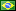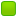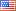###Author Topic: Other things to avoid...  (Read 638 times)

####fredericopissarra

• Full Member
•• Posts: 352
• Country:##### Other things to avoid...
« on: June 18, 2023, 02:45:48 PM »
Using floating point is one of them...

Pre-486 processors don't have an embeded 80x87 math co-processor in them. Most software at that time uses "emulated" floating point routines which are really SLOW (painfully slow - a division could take thousands of clock cycles to complete!) and, since earlier external 80x87 were available (and expensive), there were the need to use instructions like `fwait` to... well... wait the operations to complete!

`fwait`becomes obsolete on 486 and above just because the 80x87 math co-processor is embeded and it is available until now, on modern processors.

80x87 (fp87) deals with 3 structural precisions: single, double and extended, in conformance to IEEE-754 standard. In C we have `float`, `double` and `long double`, respectfully. The later (exteded) is a strange one: `float` and `double` have an "implicit" integer part set to 1 for "normalized" values (in binary), but in extended precision this bit is "excplicit", and MUST follow IEEE-754 rules: It should be 0 only for sub-normal values, where the expoent of the scale factor is minimim (E=0), otherwise, it must be 1. The only reason of existence of this structure is to accomodate 64 bits of precision in the "mantissa" (float has 24 bits, double, 53).

fp87 is still a little bit strange because don't rely on "registers", but a stack with only 8 levels, where `st(0)` is always the top of stack. Every time you push a value to the co-processor st(0) will point to that value. And, by default fp87 always deals with extended precision (you can change that in the control register).

To deal with fp87 you must master the RPN (Reverse Polish Notation) - used a lot in old HP calculators or in old languages like FORTH (FORTH is still used in Postscript!).

There is NO support for extended precision in SSE/AVX.

Another this to keep in mind is that floating point isn't "precise". This is a common mistake: The values stored in floating point structure are "fractional", but almost always "rounded". And this is easy to show... What happes if I ask you to divide some integer value by 3? What will you do? Use an integer division algorithm you learn in the school or multiply this integer value by 0.333333...? Notice n*1/3 isn't going to give you the precise answer: If you mutiply 9 by 0.33333... you'll get (if honest) 2.99999...998 (last digit rounded up at some point). This happens in binary floating point as well and it is worse!

There are lots of values that cannot be represented in floating point exactly, like 0.1, 0.2, 0.3, 0.4, 0.6, 0.7, 0.8 and 0.9, never mind the precision used... If you choose 'double precision', 0.1 is, exactly 0.1000000000000000055511151231257827021181583404541015625 (a little bit above the exact value). And gets worse: 0.3 is 0.299999999999999988897769753748434595763683319091796875, but 0.1 + 0.2 is 0.3000000000000000444089209850062616169452667236328125.

The first (0.3) is a little bit below the exact value and (0.1 + 0.2) is a little bit above. This is explained by the fact 0.1 and 0.2 are a little bit above their exact values and, when added, this small errors are added as well (and the result rounded to the nearest representable value).

Another thing: "Precision" is measured in bits, for computational purposes... So, a `double` (53 bits) has LESS precision then an `long long int` (63 bits), the same way a `float` (24 bits) has LESS precision than an `int` (31 bits).

So I recommend to avoid using floating point as far as you must...
« Last Edit: June 18, 2023, 02:51:00 PM by fredericopissarra »

####fredericopissarra

• Full Member
•• Posts: 352
• Country:##### Re: Other things to avoid...
« Reply #1 on: June 18, 2023, 03:08:16 PM »
Here's a pratical substitution of floating point calculation that will give you an EXACT value. Let's say I want the test if an image is in Widescreen aspect ration (16/9). If we get the WIDTH and HEIGHT of that image and compare to 16/9, like this:
Code: [Select]
`_Bool isWide( unsigned int w, unsigned int h ){ return (double)w / h == 16.0/9; }`Or, in asm (since this is a NASM forum) for x86-64:
Code: [Select]
`isWide:  mov       edi, edi  mov       esi, esi  cvtsi2sd  xmm0, rdi  cvtsi2sd  xmm1, rsi  divsd     xmm0, xmm1  ucomisd   xmm0, [aspect]  setnp     al  retaspect:  dq       16.0 / 9.0 `You will get false results sometimes because the constant 16/9 is 1.77777777777777767909128669998608529567718505859375 and not 1.777... (7 ad infinitum) AND because w/h will be rounded to the nearest value.

Getting back to high school (?) you can do this comparison using only integers, since (w/h == 16/9) is the same as (9*w == 16*h):
Code: [Select]
`_Bool isWide( unsigned int w, unsigned int h ){ return 9*w == 16*h; }`Or:
Code: [Select]
`isWideInt:  lea   eax, [rdi+rdi*8]  sal   esi, 4  cmp   eax, esi  sete  al  ret`Which is smaller, faster and precise.

Notice you don't need to deal with the special case where h==0 in this second function. But, of course, you'll need to deal with the case where both w and h are 0 (the same way you should deal with these cases using floating point).
« Last Edit: June 18, 2023, 03:16:48 PM by fredericopissarra »

####Frank Kotler

• NASM Developer
• Hero Member
•• Posts: 2658
• Country:##### Re: Other things to avoid...
« Reply #2 on: June 18, 2023, 07:18:36 PM »
Thank you, Fred!

I have a Celsius to/from Fahrenheit converter that will need some work. Although I'm  in no hurry...

Best,
Frank

####fredericopissarra

• Full Member
•• Posts: 352
• Country:##### Re: Other things to avoid...
« Reply #3 on: June 19, 2023, 05:28:01 PM »
Thank you, Fred!
My pleasure!
I have a Celsius to/from Fahrenheit converter that will need some work. Although I'm  in no hurry...
Well... I suggest to avoid floating point if possible, but for scientific calculations there's nothing wrong. Specially in physics, where all calculations are approximations anyway.

The floating point structure is based on the notion of "scientific notation". It is a fraction between two unsigned integers which result in a value between 1 and 2 (2 excluded), multiplied by a "scale factor" (power of 2):
Code: [Select]
`v=(-1)^S * ( 1 + F/2^(p-1) ) * 2^(E - bias)`There S is the signal bit, F is the "mantissa" (when divided by the fixed power of 2) and 2^(E-bias) is the "scale factor". S, F and E are always unsigned integers.
The scientfic notation part is in the middle, where, in binary, the integral part always have that inplicit 1.0 (F is p-1 bits long, where p is binary precision of the type - 24 for float, 53 for double).

The problem lies when someone wants to do EXACT calculations, which is, in the majority of the cases, impossible.

[]s
Fred

« Last Edit: June 19, 2023, 05:29:43 PM by fredericopissarra »